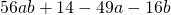Chapter 7: Factoring

# 7.2 Factoring by Grouping

First thing to do when factoring is to factor out the GCF. This GCF is often a monomial, like in the problem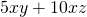where the GCF is the monomial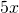, so you would have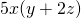. However, a GCF does not have to be a monomial; it could be a binomial. Consider the following two examples.

Example 7.2.1

Find and factor out the GCF for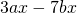.

By observation, one can see that both have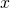in common.

This means that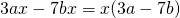.

Example 7.2.2

Find and factor out the GCF for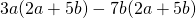.

Both have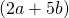as a common factor.

This means that if you factor out, you are left with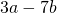.

The factored polynomial is written as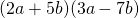.

In the same way as factoring out a GCF from a binomial, there is a process known as grouping to factor out common binomials from a polynomial containing four terms.

Find and factor out the GCF for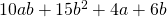.

To do this, first split the polynomial into two binomials.becomes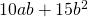and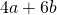.

Now find the common factor from each binomial.has a common factor of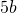and becomes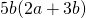.has a common factor of 2 and becomes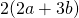.

This means that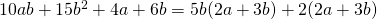.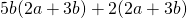can be factored as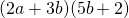.

# Questions

Factor the following polynomials.

1.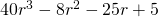2.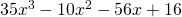3.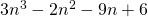4.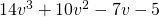5.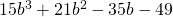6.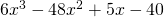7.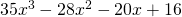8.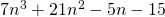9.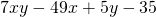10.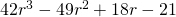11.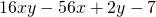12.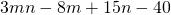13.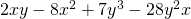14.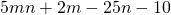15.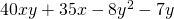16.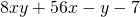17.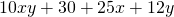18.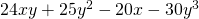19.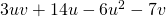20.